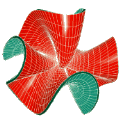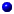Sfb 288 Differential Geometry and Quantum PhysicsResearch

The research at the Sfb falls within 5 main areas of interest:Differential Geometry and Classical Mechanics

Integrable systems in differential geometry
Experimental mathematics and visualization (some individual projects)Elliptical Operators in Geometry and Physics

Spectral properties of Dirac and Laplace operators and gauge field theory
Geometric variational problemsDiscretization in Geometry and Physics

Discrete differential geometry, quantum field theory and statistical mechanics
Lattice field theory, integrable systems and quantum symmetryAnalysis of Quantum Mechanical Systems

Semi classical analysis: Born-Oppenheimer approximation, dynamics in mean field models, the large atom limit and the adiabatic theorem
Quantum mechanical models
Spectral theory of gauge-periodic operators
Spectral invariants of singular manifolds and their deformation properties
Classical and quantum mechanical inverse problem of periodic potentialsGeometry and Algebraic Quantum Field Theory

Geometry, algebraic quantum field theory and the form factor programme

Copyright © 1999 Sfb 288, Mathematics 8-5, Strasse des 17 Juni 136, TU-Berlin, 10623 Berlin